# MP Board Class 10th Maths Solutions Chapter 3 Pair of Linear Equations in Two Variables Ex 3.2

In this article, we will share MP Board Class 10th Maths Book Solutions Pair of Linear Equations in Two Variables Ex 3.2 Pdf, These solutions are solved subject experts from the latest edition books.

## MP Board Class 10th Maths Solutions Chapter 3 Pair of Linear Equations in Two Variables Ex 3.2

Question 1.
Form the pair of linear equations in the following problems, and find their solutions graphically.
(i) 10 students of class X took part in a Mathematics quiz. If the number of girls is 4 more than the number of boys, find the number of boys and girls who took part in the quiz.
(ii) 5 pencils and 7 pens together cost ₹ 50, whereas 7 pencils and 5 pens together cost ₹ 46. Find the cost of one pencil and that of one pen.
Solution:
(i) Let the number of boys = x and Number of girls = y
∴ x + y = 10 …. (1)
Also, Number of girls = [Number of boys] + 4
∴ y = x + 4 ⇒ x – y = – 4 … (2)
Now, from the equation (1), we have :
l1 : x + y = 10 ⇒ y = 10 – x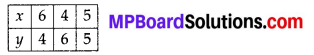And from the equation (2), we have
l2 : x – y = -4 ⇒ y = x + 4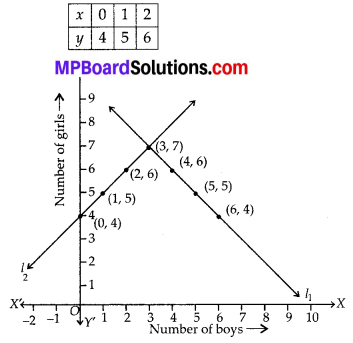The lines l1 and l2 intersects at the point (3, 7)
∴ The solution of the pair of linear equations is : x = 3, y = 7
⇒ Number of boys = 3
and number of girls = 7
Let the cost of a pencil = ₹ x
and cost of a pen = ₹ y
Since, cost of 5 pencils + Cost of 7 pens = ₹ 50
5 x + 7y = 50 … (1)
Also, cost of 7 pencils + cost of 5 pens = ₹ 46
7x + 5y = 46 …. (2)
Now, from equation (1), we have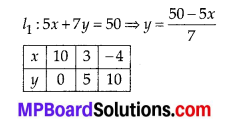And from equation (2), we have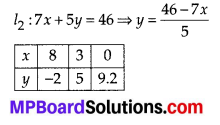Plotting the points (10, 0), (3, 5) and (-4, 10), we get a straight line l1 and plotting the points (8, -2), (3, 5) and (0, 9.2) we get a straight line l2.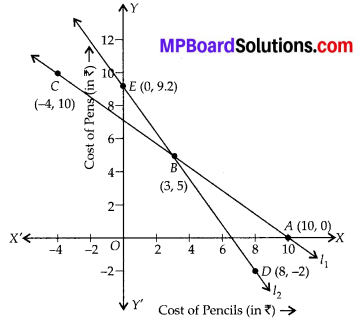These two straight lines intersects at (3, 5).
∴ Cost of a pencil = ₹ 3 and cost of a pen = ₹ 5.Question 2.
On comparing the ratios $$\frac{a_{1}}{a_{2}}, \frac{b_{1}}{b_{2}}$$ and $$\frac{c_{1}}{c_{2}}$$ find out whether the lines representing the following pairs of linear equations intersect at a point, are parallel or coincident:
(i) 5x – 4y + 8 = 0; 7x + 6y – 9 = 0
(ii) 9x + 3y + 12 = 0; 18x + 6y + 24 = 0
(iii) 6x – 3y + 10 = 0; 2x – y + 9 = 0
Solution:
Comparing the given equations with
a1x + b1y + C1 = 0, a2x + b2y + c2 = 0, we have

(i) For, 5x – 4y + 8 = 0, 7x + 6y – 9 = 0,
a1 = 5, b1 = -4, C1 = 8; a2 = 7, b2 = 6, c2 = -9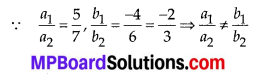So, the lines are intersecting, i.e., they intersect at a point.

(ii) For, 9x + 3y + 12 = 0,18x + 6y + 24 = 0,
a1 = 9, b1 = 3, C1 = 12; a2 = 18, b2 = 6, c2 = 24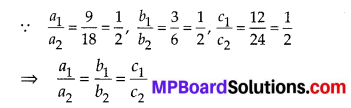So, the lines are coincident.

(iii) For, 6x – 3y +10 = 0, 2x – y + 9 = 0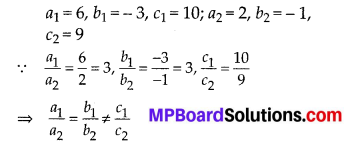So, the lines are parallel.Question 3.
On comparing the ratios $$\frac{a_{1}}{a_{2}}, \frac{b_{1}}{b_{2}}$$ and $$\frac{c_{1}}{c_{2}}$$, find out whether the following pair of linear equations are consistent, or inconsistent.
(i) 3x + 2y = 5; 2x – 3y = 7
(ii) 2x – 3y = 8; 4x – 6y = 9
(iii) $$\frac{3}{2} x+\frac{5}{3} y$$ = 7; 9x-10y = 14
(iv) 5x – 3y = 11; -10x + 6y = -22
Solution:
Comparing the given equations with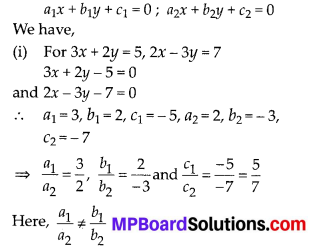So, lines are intersecting i.e., they intersect at a point.
∴ It is consistent pair of equations.

(ii) For 2x – 3y = 8, 4x – 6y = 9, 2x – 3y – 8 = 0 and 4x – 6y – 9 = 0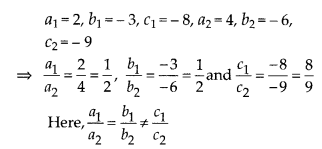So, lines are parallel i.e., the given pair of linear equations has no solution.
∴ It is inconsistent pair of equations.

(iii)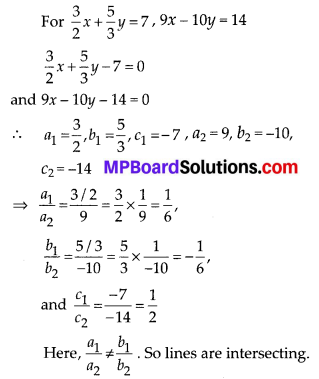⇒ The given pair of linear equations has exactly one solution.
∴ It is a consistent pair of equations.

(iv)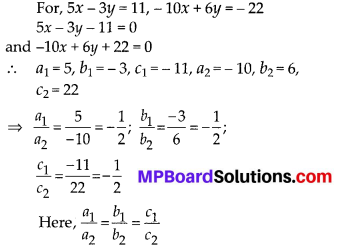So, lines are coincident.
⇒ The given pair of linear equations has infinitely many solutions.
Thus, it is consistent pair of equations

(v)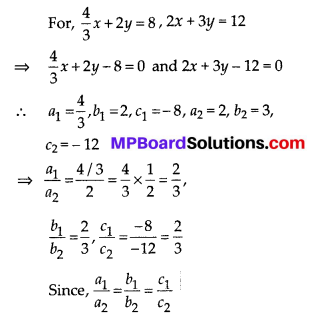So, the lines are coincident.
⇒ The given pair of linear equations have infinitely many solutions.
Thus it is consistent pair of equations.Question 4.
Which of the following pairs of linear equations are consistent / inconsistent? If consistent, obtain the solution graphically:
(i) x + y = 5; 2x + 2y = 10
(ii) x – y = 8; 3x – 3y = 16
(iii) 2x + y – 6 = 0; 4x – 2y – 4 = 0
(iv) 2x – 2y – 2 = 0; 4x – 4y – 5 = 0
Solution:
(i) For, x + y = 5, 2x + 2y = 10 ⇒ x + y – 5 = 0 and 2x + 2y – 10 = 0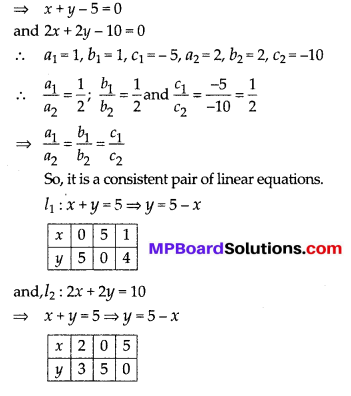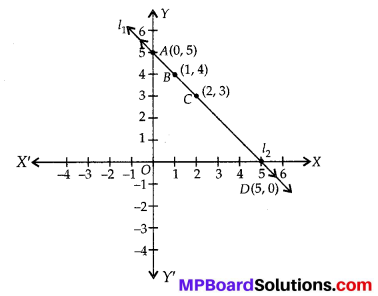So, lines l1 and l2 are coinciding. i.e., They have infinitely many solutions.

(ii) For, x – y = 8,
3x – 3y = 16
⇒ x – y – 8 = 0
and 3x – 3y = 16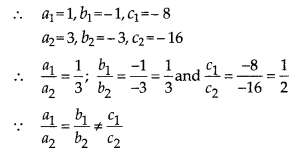so, line are parallel
∴ The pair of linear equations are inconsistent

(iii) For 2x + y – 6 = 0, 4x – 2y – 4 = 0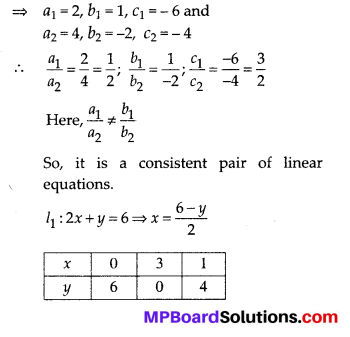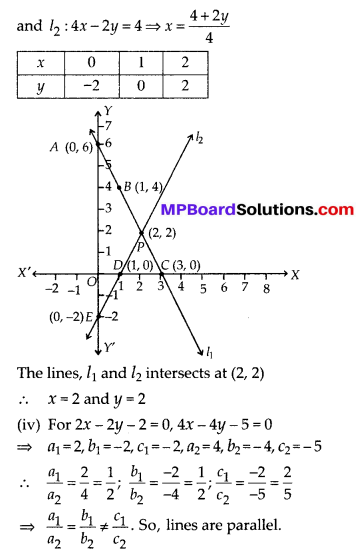The pair of linear equations are inconsistentQuestion 5.
Half the perimeter of a rectangular garden, whose length is 4 m more than its width, is 36 m. Find the dimensions of the garden.
Solution:
Let the width of the garden = x m
and the length of the garden = y m
According to question,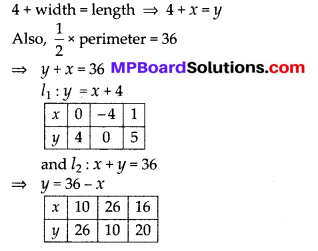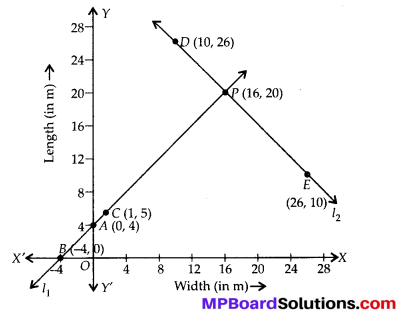The lines l1 and l2 intersect at (16, 20).
∴ x = 16 and y = 20
So, Length = 20m, and Width = 16mQuestion 6.
Given the linear equation 2x + 3y – 8 = 0, write another linear equation in two variables such that the geometrical representation of the pair so formed is:
(i) intersecting lines
(ii) parallel lines
(iii) coincident lines
Solution:
Let the pair of linear equations be 2x + 3y – 8 = 0
⇒ a1 = 2, b1 = 3 and C1 = -8 and a2x + b2y + c2 = 0.

(i) For intersecting lines, we have: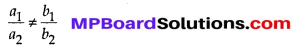∴ We can have a2 = 3, b2 = 2 and c2 = – 7
∴ The required equation can be 3x + 2y – 7 = 0

(ii) For parallel lines, $$\frac{a_{1}}{a_{2}}=\frac{b_{1}}{b_{2}} \neq \frac{c_{1}}{c_{2}}$$
∴ Any line parallel to 2x + 3y – 8 = 0, can be taken as 2x + 3y -12 = 0

(iii) For coincident lines, $$\frac{a_{1}}{a_{2}}=\frac{b_{1}}{b_{2}}=\frac{c_{1}}{c_{2}}$$
∴ Any line parallel to 2x + 3y – 8 = 0 can be 2(2x + 3y – 8 = 0)
⇒ 4x + 6y – 16 = 0Question 7.
Draw the graphs of the equations x – y + 1 = 0 and 3x + 2y – 12 = 0. Determine the coordinates of the vertices of the triangle formed by these lines and the x-axis, and shade the triangular region.
Solution:
Since, we have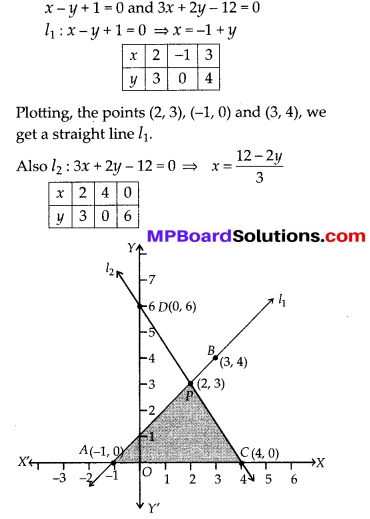The lines l1 and l2 intersect at (2, 3). Thus, co-ordinates of the vertices of the shaded triangular region are (4, 0), (-1, 0) and (2, 3).